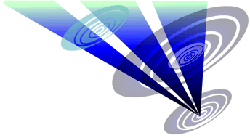Reconstruction of the 2015 Etchilhampton (2) formation 1.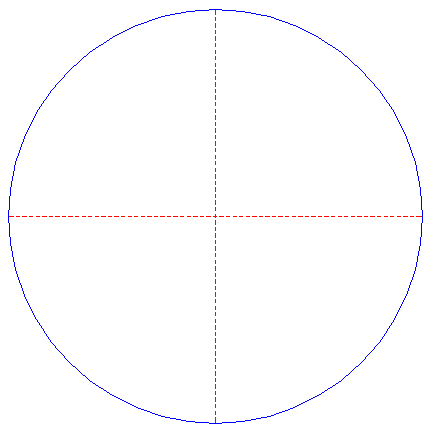Draw a circle. Draw the horizontal and vertical centerlines. 2.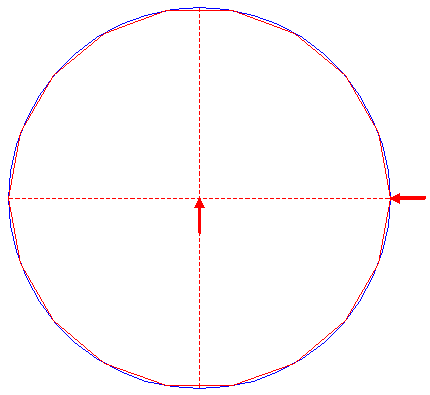Construct the inscribed regular 18-sided polygon of circle 1, pointing to the right. 3.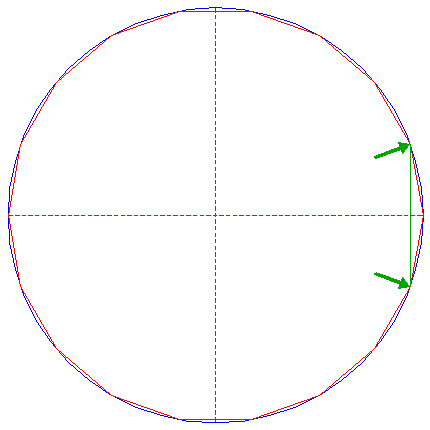Draw the connecting line between the righthand angular points of polygon 2 just above and below the horizontal centerline. 4.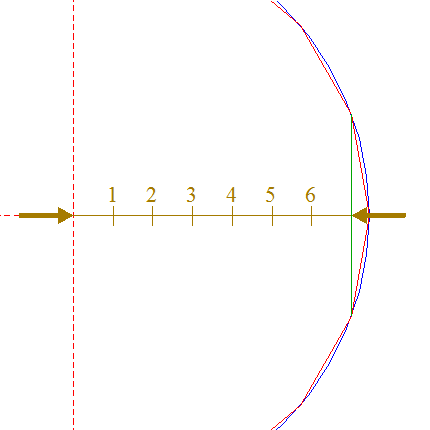Draw the connecting line between the center of circle 1 and the intersection of line 3 and the horizontal centerline. Divide this line into seven equal parts, and number the nodes from left to right 1 - 6. 5.Construct a circle concentric to circle 1, passing through node 4 nr. 1. 6.Construct a circle concentric to circle 1, passing through node 4 nr. 2. 7.Construct a circle concentric to circle 1, passing through node 4 nr. 3. 8.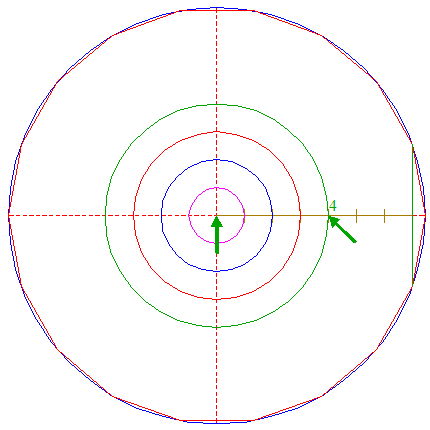Construct a circle concentric to circle 1, passing through node 4 nr. 4. 9.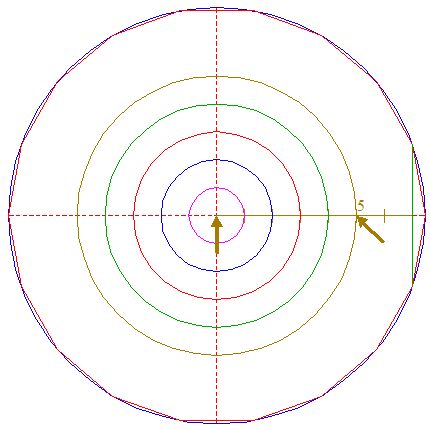Construct a circle concentric to circle 1, passing through node 4 nr. 5. 10.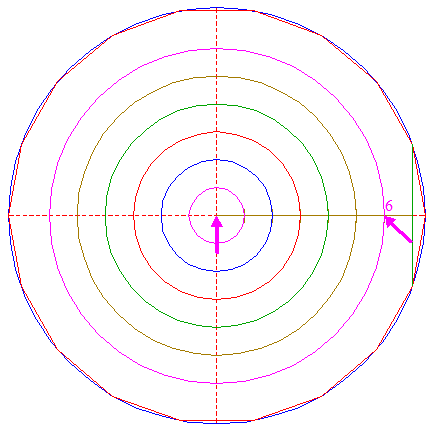Construct a circle concentric to circle 1, passing through node 4 nr. 6. 11.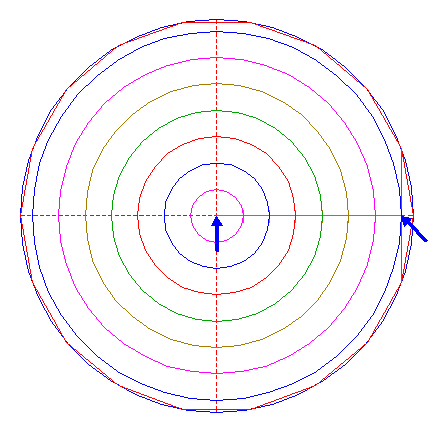Construct a circle concentric to circle 1, passing through the intersection of line 3 and the horizontal centerline. 12.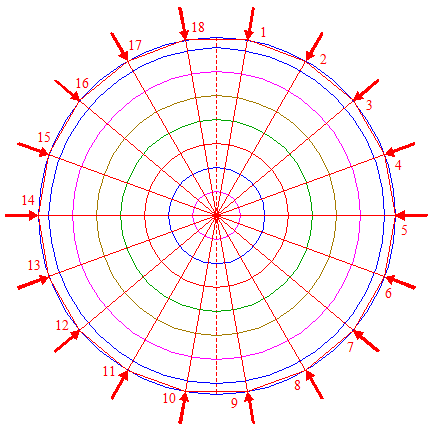Draw the eighteen rays of polygon 2, from the center of circle 1 to the angular points. Number the rays clockwise 1 - 18, starting at the uppermost righthand angular point. 13.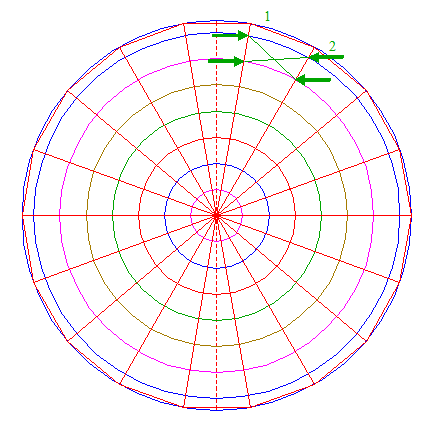Draw two connecting lines, one between the intersection of ray 12 nr. 1 and circle 11 and the intersection of ray 12 nr. 2 and circle 10, the other between the intersection of ray 12 nr. 1 and circle 10 and the intersection of ray 12 nr. 2 and circle 11. 14.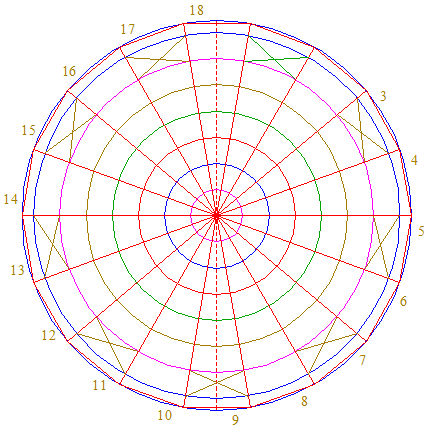Repeat step 13 eight times, with respect to pairs of rays 12 nrs. 3 and 4, 5 and 6, 7 and 8, 9 and 10, 11 and 12, 13 and 14, 15 and 16, and 17 and 18. 15.Draw two connecting lines, one between the intersection of ray 12 nr. 2 and circle 10 and the intersection of ray 12 nr. 3 and circle 9, the other between the intersection of ray 12 nr. 2 and circle 9 and the intersection of ray 12 nr. 3 and circle 10. 16.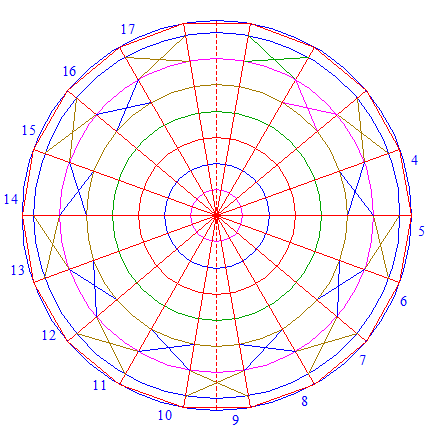Repeat step 15 seven times, with respect to pairs of rays 12 nrs. 4 and 5, 6 and 7, 8 and 9, 10 and 11, 12 and 13, 14 and 15, and 16 and 17. 17.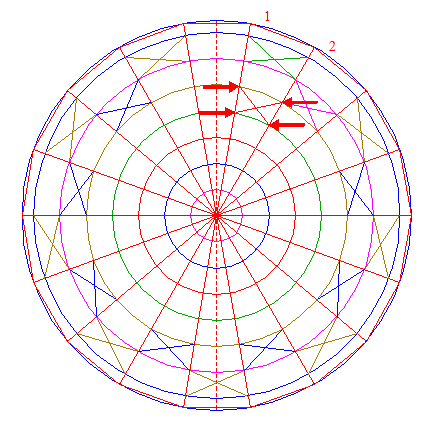Draw two connecting lines, one between the intersection of ray 12 nr. 1 and circle 9 and the intersection of ray 12 nr. 2 and circle 8, the other between the intersection of ray 12 nr. 1 and circle 8 and the intersection of ray 12 nr. 2 and circle 9. 18.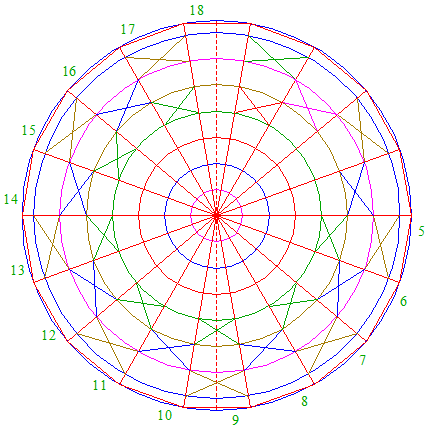Repeat step 17 seven times, with respect to pairs of rays 12 nrs. 5 and 6, 7 and 8, 9 and 10, 11 and 12, 13 and 14, 15 and 16, and 17 and 18. 19.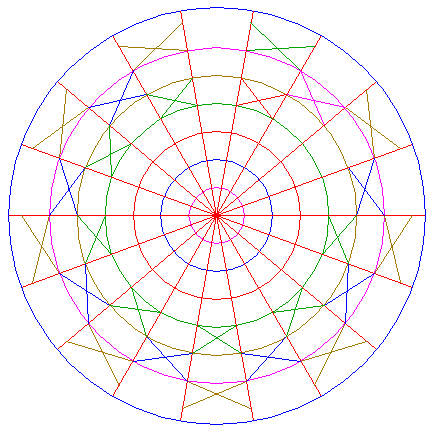Circles 1, 5, 6, 7, 8, 9, and 10, and lines 12, 13, 14, 15, 16, 17, and 18, are used for the final reconstruction. 20.Remove all parts not visible within the formation itself. 21.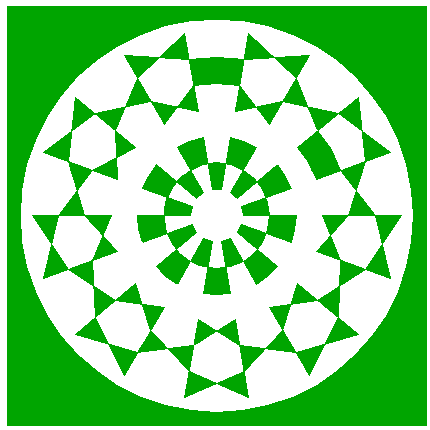Colour all areas corresponding to standing... 22.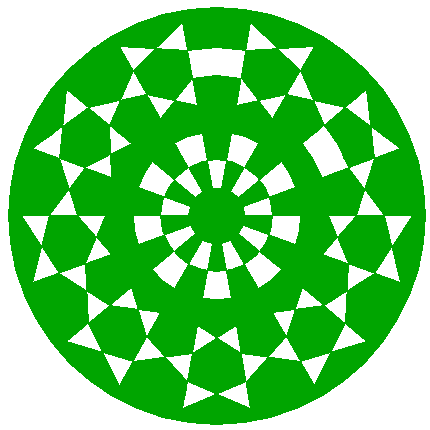...or to flattened crop, and finish the reconstruction of the 2015 Etchilhampton (2) formation. 23.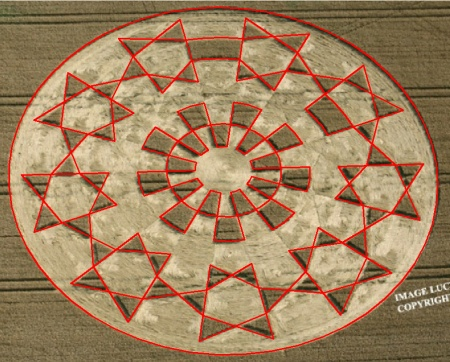The final result, matched with the aerial image.